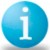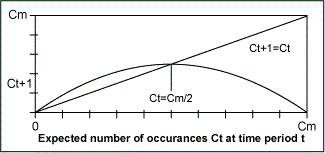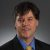# A Simple Model of a Variance Stable Process

ByJohn J. Hickey

Most fairly accurate descriptions of equipment and/or process lifetimes assume that failure rates follow a three period I II III “bathtub-curve pattern” where failures/errors:

I – Decrease during the debugging or improvement time period.

II – Remain relatively constant and at their lowest levels during the normal equipment or process operating period.

III – Increase during the wearout time period.

Scientific studies of limit based natural or complex growth patterns also suggest that many processes are inherently non-linear and subject to chaotic tendencies. The logistics map3 or parabola Xt + 1 = RXt (1-Xt) where Xt + 1 the measure of the next generation is a function of the present measure Xt, R is the growth factor and t is a discrete time variable is a simple model for these processes. When the growth factor R falls within the range of 1 < R < 3 the process is stable. For R = 2, the time series iterates Xt = X1 X2 X3… converge to the constant value Xc =.5 which can be easily demonstrated (see Table 1) through the use of an Excel spreadsheet or pocket calculator.

 Table 1: Logistics Map – Xt + 1 = Rxt (1-Xt) Calculated Iterates Process Category Unstable Decreasing Stable Constant Unstable Oscillation Unstable Oscillation Unstable Chaotic R = 1 2 3 3.24 3.8 X0 0.800 0.800 0.800 0.800 0.800 X1 0.160 0.320 0.480 0.518 0.608 X2 0.134 0.435 0.749 0.809 0.906 X3 0.116 0.492 0.564 0.501 0.325 X4 0.103 0.500 0.738 0.810 0.833 X5 0.092 0.500 0.581 0.499 0.528 X6 0.084 0.500 0.730 0.810 0.947 X7 0.077 0.500 0.591 0.499 0.191 X8 0.071 0.500 0.725 0.810 0.587 X9 0.066 0.500 0.598 0.498 0.921

The critical growth factor value Rcr = 3.24 (51/2 +1 ) in Table 1 signals the start of chaotic instability in this model process and for R = 3.8 the instability is clearly evident.

Handpicked Content:   Drive Improvements in Outbound Telesales with Lean Six Sigma: Part 2 of 2

### Process Variance Stability

If we assume that variance (Vt) of a process during its lifetime varies between zero and some maximum acceptable value Vm, the application of the logistics parabola model to the process results in the iterate expression Vt + 1 = RVt (Vm – Vt). In this case the process is stable1 within the growth factor R range of 1/Vm < R < 3/Vm. Also, the process attains super-stability or constancy when its variance equals one half of the maximum acceptable value (Vt = Vm/2) and when R = 2/Vm. This is illustrated for a process with a maximum-allowed variance of Vm= 9 (standard deviation =3) in Table 2.

 Table 2: Super-stable Process Variance Map – Vt + 1 = RVt (9 – Vt) Variance Category Unstable Decreasing Super-stable Constant Unstable Oscillation Unstable Oscillation Unstable Chaotic R = 0.111 0.222 0.333 0.360 0.422 V0 4.50 4.50 4.50 4.50 4.50 V1 2.25 4.50 6.74 7.29 8.55 V2 1.68 4.50 5.07 4.49 1.64 V3 1.37 4.50 6.64 7.29 5.09 V4 1.16 4.50 5.22 4.49 8.40 V5 1.01 4.50 6.57 7.29 2.13 V6 0.89 4.50 5.32 4.49 6.18 V7 0.80 4.50 6.52 7.29 7.35 V8 0.73 4.50 5.38 4.49 5.12 V9 0.67 4.50 6.48 7.29 8.39Super-Stable Poisson Distribution – Ct + 1 = 2 / CmCt (Quadratic Map)

The values of R in Table 2 are obtained by scaling the R values of Table I by 1/Vm = 1/9. For example, R = 2/9= .222 is the super-stable growth factor and Rcr = 3.24/9 =.36 is the critical factor. In the case of Poisson-distributed processes, the expected number of occurrences C = NP (large N, small fraction P of occurrence) is both the variance and mean of the distribution. A conditional Poisson process that conformed to this simple non-linear model has the variance Ct + 1 = RCt (Cm -Ct) and would be stable in growth rate range 1/Cm < R < 3/Cm where Cm is the specified maximum number of occurrences. When Ct = Cm/2 and R = 2/Cm the process is super-stable1 and ideally Poisson because the expected number of occurrences Co = C1 = C2….= Ct = Ct + 1 remain constant and are time independent over the operating lifetime of the process. This condition of super-stability is analogous to “States of Equilibrium” in Statistical Mechanics2 and is illustrated by the Ct + 1 = Ct intersecting line of above Figure I quadratic map.

Handpicked Content:   Drive Improvements in Outbound Telesales with Lean Six Sigma: Part 1 of 2

The hypothetical model is suggestive of an ideal, super-stable six sigma process with an expected Poisson failure no of C = 1.7 PPM (N= 106, P= 1.7 x 10-6), maximum failure number of Cm = 3.4 PPM and growth factor that has the value R = .60.

A real-world stable process would of course exhibit random fluctuations in variance which would not be strictly deterministic. However, as it ages or deteriorates and becomes unstable some deterministic chaos may be present and evident by an oscillatory pattern of variance (e.g., machine tool wear). If a process is stable with a relatively constant variance and it meets requirements (in my opinion) it does not need to be fixed.

### Notes and References

1. |F'(Vt)| < 1 is the first derivative criteria for the stability of the fixed points Vt = 0, Vt = Vm – 1/R in the Variance quadratic map Vt + 1 = R Vt (Vm-Vt). Since F'(Vt) = R (Vm-2Vt), the stability ranges for the fixed points Vt = 0, Vt = Vm – 1/R calculate as R < 1/Vm and 1/Vm < R < 3/Vm respectively. Vt = Vm/2 is the value of the non zero fixed point at the growth rate R = 2 /Vm and F'(Vt) = 0 when this occurs. Therefore, a super-stable Poisson process Variance Ct would be “ideally Poisson” because its expected number of occurrences Ct = Ct+1…remain constant during successive time periods. The oscillatory behavior of the logistic parabola iterates in the unstable growth rate region R ≥ 3 is known as 2n period doubling. It is represented mathematically by the composite function expression Fn (X0) = X0 where n is the number of cycles or iterations required for a repetition of the point X0. The cycles or “splittings” increase as the associated growth rates R1..R2.. become larger. Chaos and infinite period doubling occurs with R > 3.6/Vm. For the 2-cycle period F2 (X0) = X0 and super-stable fixed point X0 =.5, the growth rate for Vm = 1 is Rc = 51/2 + 1.
2. E.C. Andrews, Equilibrium Statistical Mechanics, John Wiley & Sons Inc., 1975 defines a state of equilibrium as one in which the information we have about the system it has reached a time -independent minimum. In the Chapter 7 section on ensembles with minimum information he proves that a maximum ignorance (lack of assigned causes) about the system exists when the state probabilities are equal.
3. The technical literature on non-linear dynamics, logistic equations, quadratic maps, fixed-point stability, period doubling and chaos is extensive.
##### John J. Hickey

1.•